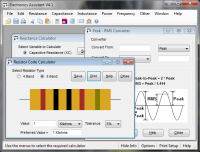# Capacitor Code Calculator

These calculators can convert the 3-digit value codes and alphabetical tolerance codes found on some capacitors into the corresponding value and vice-versa. Both parts of each calculator work separately - you do not have to enter both value code and tolerance code. Details of capacitor markings can be found in the technical data section.

#### Convert Codes to Values:

 Enter 3-digit Value Code: Select Tolerance Code: C D F G J K M Z Capacitance = ? Tolerance = +/- 20%

#### Convert Values to Codes:

 Enter Capacitance: Microfarads Nanofarads Picofarads Select Tolerance: +/- 0.25pF +/- 0.5pF +/- 1% +/- 2% +/- 5% +/- 10% +/- 20% -20% +80% Code = ? Tolerance Code = M

# If you found this calulator useful you should try Electronics Assistant!• All the online calculators and more in a stand-alone application
• Converts Resistor & Inductor colour codes, calculates LED series resistors, capacitance units, series / parallel resistors & capacitors, frequency, reactance & more
• Calculation of nearest preferred resistor values with a choice of 5 series from E12 to E192
• Print & save calculation results